## Exercises

1. Characterize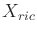for the case of a point mass in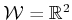, with each coordinate modeled as a double integrator. Assume that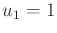and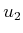may take any value in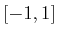. Determinefor:
1. A point obstacle at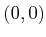in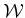.
2. A segment from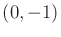to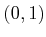in.
Characterize the solutions in terms of the phase variables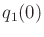,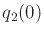,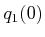, and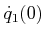.
2. Extending the double integrator:
1. Develop a lattice for the triple integrator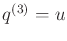that extends naturally from the double-integrator lattice.
2. Describe how to develop a lattice for higher order integrators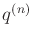for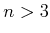.
3. Make a figure similar to Figure 14.6b, but for three stages of the Reeds-Shepp car.
4. Determine expressions for the upper and lower boundaries of the time-limited reachable sets shown in Figure 14.14. Express them as parabolas, with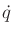as a function of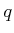.
5. A reachability graph can be made by rolling'' a polyhedron in the plane. For example, suppose a solid, regular tetrahedron is placed on a planar surface. Assuming high friction, the tetrahedron can be flipped in one of four directions by pushing on the top. Construct the three-stage reachability graph for this problem.
6. Construct a four-stage reachability graph similar to the one shown in Figure 14.6b, but for the case of a differential drive robot modeled by (13.17). Use the three actions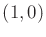,, and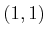. Draw the graph in the plane and indicate the configuration coordinates of each vertex.
7. Section 14.2.2 explained how resolution-complete algorithms exist for planning under differential constraints. Suppose that in addition to continuous state variables, there are discrete modes, as introduced in Section 7.3, to form a hybrid system. Explain how resolution-complete planning algorithms can be developed for this case. Extend the argument shown in Figure 14.7.

Implementations

8. Compare the performance and accuracy of Euler integration to fourth-order Runge-Kutta on trajectories generated for a single, double, and triple integrator. For accuracy, compare the results to solutions obtained analytically. Provide recommendations of which one to use under various conditions.
9. Improve Figure 14.13 by making a plot of the actual trajectories, which are parabolic in most cases.
10. In Figure 14.13, the state trajectory segments are longer as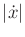increases. Develop a lattice that tries to keep all segments as close to the same length as possible by reducing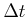asincreases. Implement and experiment with different schemes and report on the results.
11. Develop an implementation for computing approximately time-optimal state trajectories for a point mass in a 2D polygonal world. The robot dynamics can be modeled as two independent double integrators. Search the double-integrator lattice in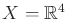to solve the problem. Animate the computed solutions.
12. Experiment with RDT methods applied to a spacecraft that is modeled as a 3D rigid body with thrusters. Develop software that computes collision-free trajectories for the robot. Carefully study the issues associated with choosing the metric on.
13. Solve the problem of optimally bringing the Dubins car to a goal region in a polygonal world by using value iteration with interpolation.
14. Select and implement a planning algorithm that computes pushing trajectories for a differential drive robot that pushes a box in a polygonal environment. This was given as an example of a nonholonomic system in Section 13.1.3. To use the appropriate constraints on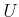, see .
15. Select and implement a planning algorithm that computes trajectories for parking a car while pulling a single trailer, using (13.19). Make an obstacle region inthat corresponds to a tight parking space and vary the amount of clearance. Also, experiment with driving the vehicle through an obstacle course.
16. Generate a 3D rendering of reachability graphs for the airplane model in (13.20). Assume that in each stage there are nine possible actions, based on combinations of flying to the right, left, or straight and decreasing, increasing, or maintaining altitude.
17. Implement the dynamic programming algorithm shown in Figure 14.27 for the two-link manipulator model given in Example 13.13.
18. Implement the bang-bang algorithm shown in Figure 14.28 for the two-link manipulator model given in Example 13.13.
19. For the Dubins car (or another system), experiment with generating a search graph based on Figure 14.7 by alternating between various step sizes. Plot in the plane, the vertices and state trajectories associated with the edges of the graph. Experiment with different schemes for generating a resolution-complete search graph in a rectangular region and compare the results.
20. Use value iteration with interpolation to compute the optimal cost-to-go for the Reeds-Shepp car. Plot level sets of the cost-to-go, which indicate the time-limited reachable sets. Compare the result to Figure 14.4.

Steven M LaValle 2020-08-14